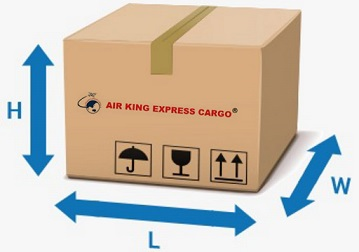Email:cs@airkingexpress.net

Call Us:9322033242/9323236687

###### Volumetric Weight explained

The cost of your parcel can be affected by the amount of space that it occupies on an aircraft, rather than the actual weight.
Shipment charge is calculated by weight (actual or dimensional whichever is greater). The Rapid Parcel booking system
automatically calculates these so no need to worry - this section is simply to aid your understanding of the industry.

###### Calculating the Volumetric Weight of Your Shipment

The conversion factor used for calculating the volumetric weight of your shipment will depend on whether your shipment is
domestic or international.

The domestic calculation is typically:

length x width x height (cm) / 4000*

For time definite and International shipments the conversion factor is 5000. The formula for calculating volumetric weight is:

length x width x height (cm) / 5000**

________________________________________________________________________________________

To calculate volumetric weight enter